# sklearn机器学习：随机森林学习与调参

33 篇文章 3 订阅

1. 集成算法介绍
2. 随机森林分类器
3. 随机森林回归器
4. 随机森林调参实例

## 集成学习

（2）、基学习器之间不存在强依赖关系，可同时生成的并行化方法，代表是Bagging和随机森林。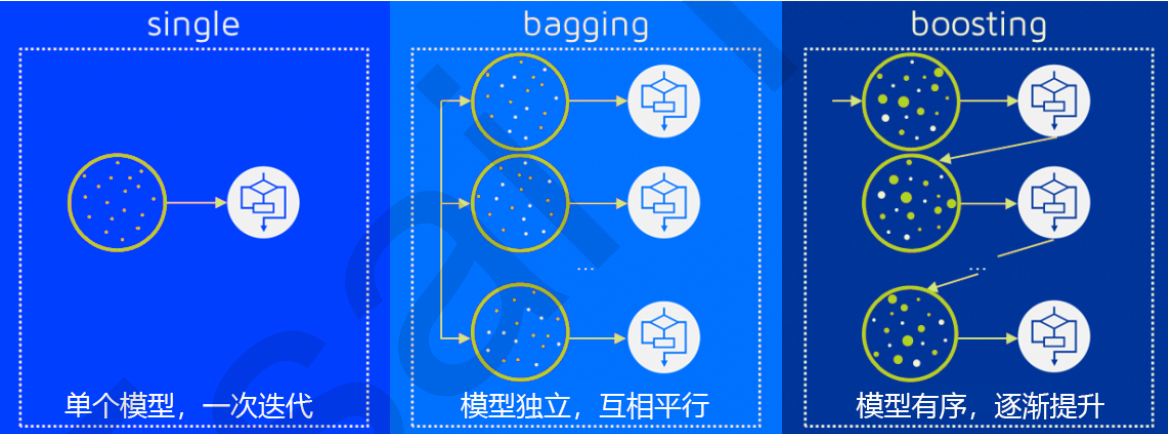sklearn中的集成学习模块如下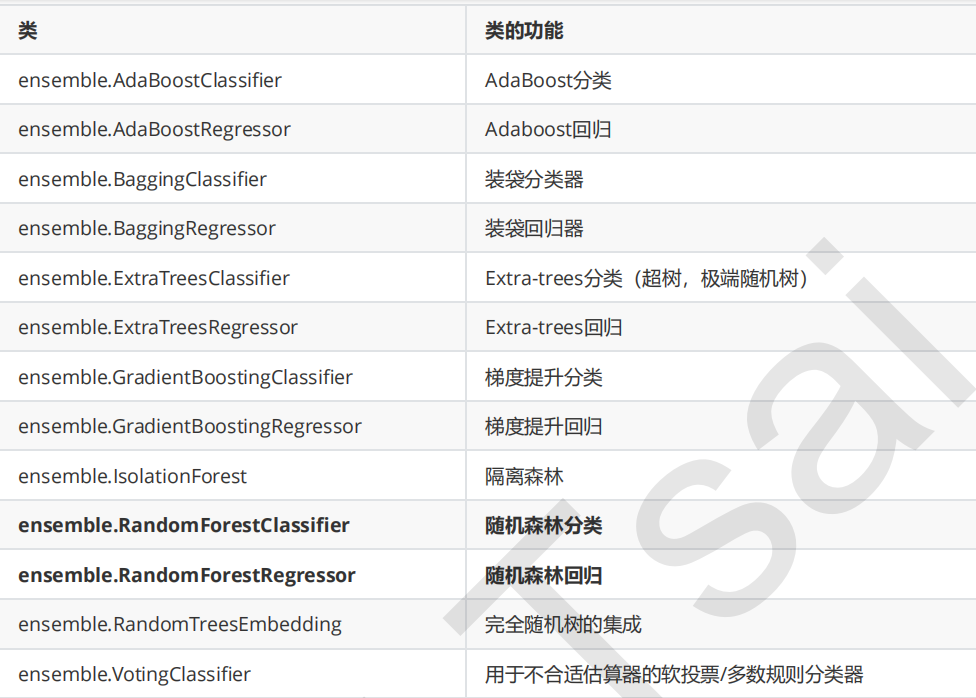## 随机森林分类

class sklearn.ensemble.RandomForestClassifier (n_estimators=’10’, criterion=’gini’, max_depth=None,
min_samples_split=2, min_samples_leaf=1, min_weight_fraction_leaf=0.0, max_features=’auto’,
max_leaf_nodes=None, min_impurity_decrease=0.0, min_impurity_split=None, bootstrap=True, oob_score=False,
n_jobs=None, random_state=None, verbose=0, warm_start=False, class_weight=None)


#一、在红酒数据集上看看决策树和随机森林的效果

#导入需要的包
import numpy as np
import pandas as pd
import matplotlib.pyplot as plt
from sklearn.ensemble import RandomForestClassifier
from sklearn.tree import DecisionTreeClassifier
from sklearn.model_selection import train_test_split
#加载到红酒数据集   是个字典
#实例化决策树模型和随机森林模型
clf = DecisionTreeClassifier(random_state=15)
rfc = RandomForestClassifier(random_state=15)
#训练集和测试集的划分
Xtrain,Xtest,Ytrain,Ytest = train_test_split(wine.data,wine.target,test_size=0.3)
#进行模型的拟合
clf.fit(Xtrain,Ytrain)
rfc.fit(Xtrain,Ytrain)
#获取模型的评分
score_1 = clf.score(Xtest,Ytest)
score_2 = rfc.score(Xtest,Ytest)
print("决策树:评分{}".format(score_1),"随机森林:评分{}".format(score_2))


#二、决策树和随机森林在一组交叉验证下的对比
from sklearn.model_selection import cross_val_score
clf = DecisionTreeClassifier(random_state=15)
rfc = RandomForestClassifier(random_state=15)
#进行交叉验证 数据分成的是10等分
score_1 = cross_val_score(clf,wine.data,wine.target,cv=10)
score_2 = cross_val_score(rfc,wine.data,wine.target,cv=10)

plt.plot(range(1,11),score_1,label="Decision Tree")
plt.plot(range(1,11),score_2,label="RandomForest")
plt.legend()
plt.show()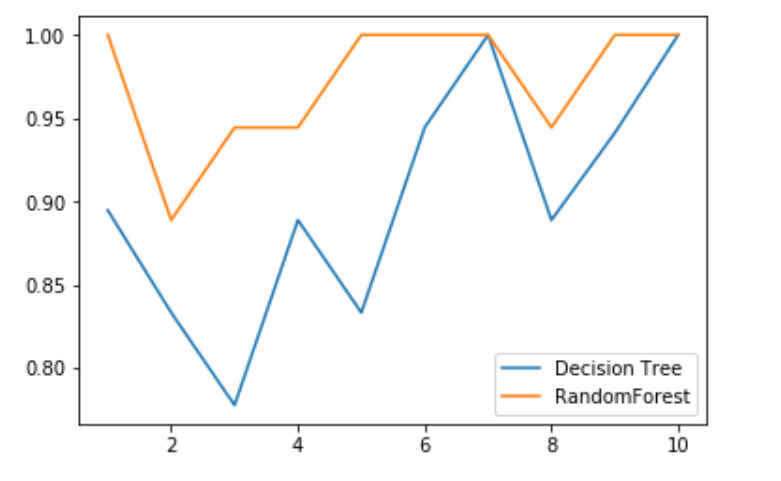#三、随机森林和决策树在十组交叉验证下的效果对比
clf_l = []
rfc_l = []
for i in range(10):
clf = DecisionTreeClassifier()
rfc = RandomForestClassifier()
l1 = cross_val_score(clf,wine.data,wine.target,cv=5).mean()
l2 = cross_val_score(rfc,wine.data,wine.target,cv=5).mean()
clf_l.append(l1)
rfc_l.append(l2)
plt.plot(range(1,11),clf_l,label="Decision Tree")
plt.plot(range(1,11),rfc_l,label="RandomForest")
plt.legend()
plt.show()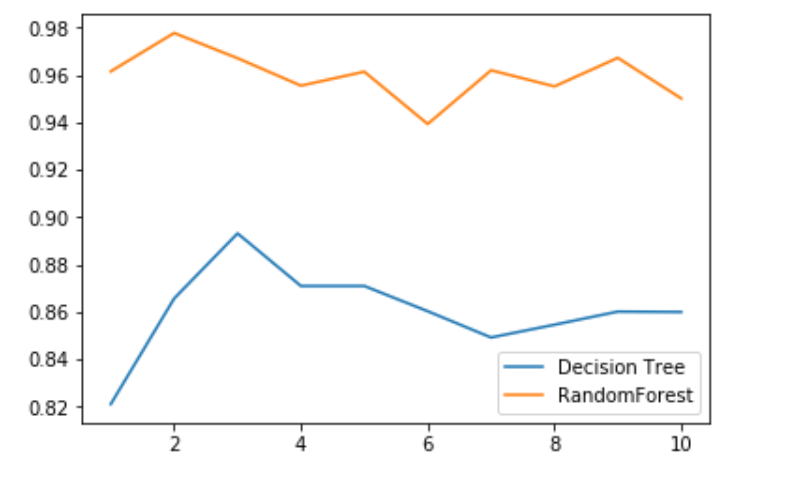#四、n_estimators的学习曲线2分多钟
rfc_l = []
for i in range(200):
rfc = RandomForestClassifier(n_estimators=i+1)
rfc_s = cross_val_score(rfc,wine.data,wine.target,cv=10).mean()
rfc_l.append(rfc_s)
print(max(rfc_l),rfc_l.index(max(rfc_l))+1)
plt.plot(range(1,201),rfc_l,label='随机森林')
plt.legend()
plt.show()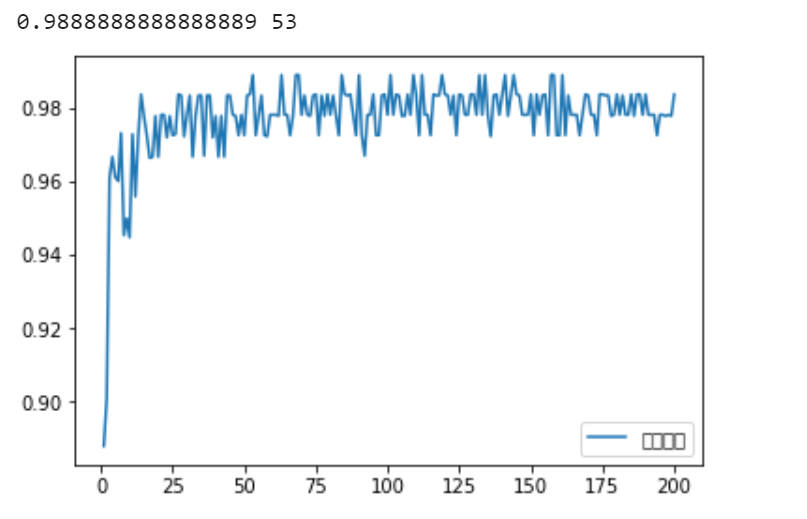plt.rcParams['font.sans-serif']=['SimHei'] #用来正常显示中文标签
plt.rcParams['axes.unicode_minus']=False #用来正常显示负号


ϵ = ∑ i = 13 25 C 25 i ϵ i ( 1 − ϵ ) 25 − i = 0.00036904803455582827 \epsilon=\sum\limits_{i=13}^{25}C_{25}^{i}\epsilon^i(1-\epsilon)^{25-i}=0.00036904803455582827

#五、计算误判率
from scipy.special import comb
np.array([comb(25,i)*(0.2**i)*(0.8**(25-i)) for i in range(13,26)]).sum()


#六、random_state在决策树中控制一棵树，在随机森林中是控制一片森林
rfc = RandomForestClassifier(random_state=10)
rfc.fit(Xtrain,Ytrain)
#使用estimators_属性查看森林中树的情况   可以看单独的一棵树，甚至可以看到参数  决策树是不可以的
#rfc.estimators_.random_state
for i in range(len(rfc.estimators_)):
print(rfc.estimators_[i].random_state)  #每次都是不变的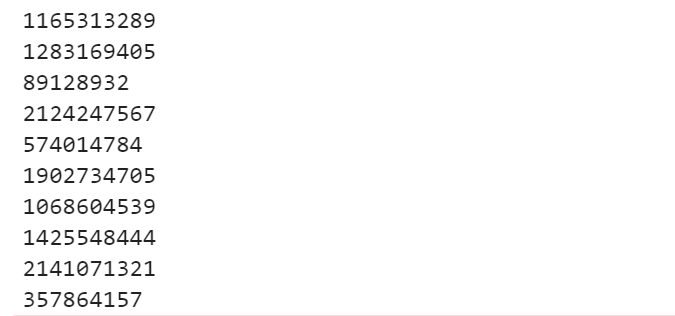bootstrap参数默认True，代表采用这种有放回的随机抽样技术。通常也不会设置为False。

#七、oob_score_属性   参数oob_score=True表示使用袋外数据进行测试
rfc = RandomForestClassifier(random_state=20,oob_score=True)
rfc.fit(wine.data,wine.target)
rfc.oob_score_   #查看分数


## 随机森林回归

#八、随机森林回归 和分类基本一样

from sklearn.datasets import load_boston
from sklearn.ensemble import RandomForestRegressor
#使用波士顿房价数据集
frr = RandomForestRegressor(random_state=20)
#评分标准是R平方，如果想使用MSE那么就使用交叉验证好了
frr_s = cross_val_score(frr,boston.data,boston.target,cv=10,scoring="neg_mean_squared_error")


#九、用随机森林回归来填补缺失值
from sklearn.impute import SimpleImputer
import numpy as np
import pandas as pd
import matplotlib.pyplot as plt
from sklearn.ensemble import RandomForestRegressor
from sklearn.model_selection import cross_val_score

#boston.data.shape   #(506, 13)  =6578个数据

X_mat,Y_mat = boston.data, boston.target
X_ori,Y_ori = X_mat.copy(), Y_mat.copy()#记住要用copy不能直接赋值，负责最后X_ori就变了
samples_ = X_mat.shape
features_ = X_mat.shape
#让一半数据缺失
missing_rate = 0.5
missing_num = int(round(samples_*features_*missing_rate))

rng = np.random.RandomState(10)
missing_rec = rng.randint(0,samples_,missing_num)
missging_features = rng.randint(0,features_,missing_num)
X_mat[missing_rec,missging_features] = np.nan
X_mat = pd.DataFrame(X_mat)

#使用均值填充缺失值
strategy_mean = SimpleImputer(missing_values=np.nan,strategy='mean')
X_mat_mean = strategy_mean.fit_transform(X_mat)
#使用0填补缺失值
strategy_0 = SimpleImputer(missing_values = np.nan, strategy='constant', fill_value= 0)
X_mat_0 = strategy_0.fit_transform(X_mat)
#使用回归填补缺失值

X_mat_reg = X_mat.copy()
sorted_index = np.argsort(X_mat_reg.isnull().sum(axis=0))

for i in sorted_index:
df = X_mat_reg
Y_fill = df.iloc[:,i]#获取需要填补的列 行对行进行拼接
new_matrix = pd.concat([df.iloc[:,df.columns != i],pd.DataFrame(Y_mat)],axis=1)#新的特征矩阵
#缺失的位置填补0 此时得到了numpy类型的数组ndarray
new_matrix = SimpleImputer(missing_values=np.nan,strategy='constant',fill_value=0).fit_transform(new_matrix)
#获取新的训练集和测试集
Ytrain = Y_fill[Y_fill.notnull()]
Ytest = Y_fill[Y_fill.isnull()]
Xtrain = new_matrix[Ytrain.index,:]
Xtest = new_matrix[Ytest.index]
#进行拟合
rfr = RandomForestRegressor(n_estimators=100)
rfr.fit(Xtrain,Ytrain)
Y_predict = rfr.predict(Xtest)
#进行填补
X_mat_reg.loc[X_mat_reg.iloc[:,i].isnull(),i] = Y_predict

#进行建模
mse = []
for i in [X_ori,X_mat_mean,X_mat_0,X_mat_reg]:
rfr = RandomForestRegressor(n_estimators=100)
score_ = cross_val_score(rfr,i,Y_ori,cv = 10,scoring="neg_mean_squared_error").mean()
mse.append(score_*-1)
print(mse)


[21.964833688980388, 31.832657715450978, 37.473234113960785, 18.107877085411765]


## 随机森林调参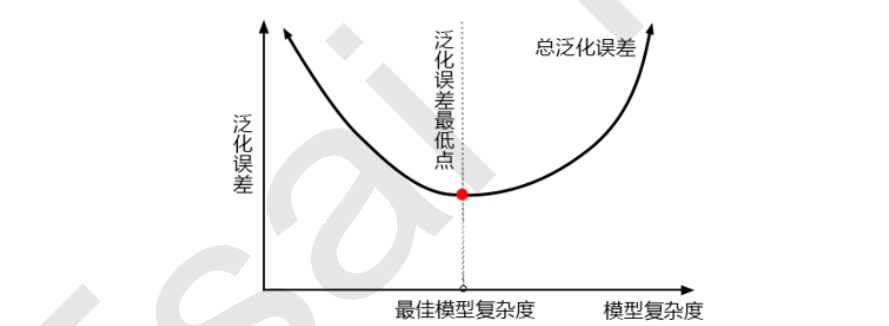1. 模型太复杂或者太简单，都会让泛化误差高，我们追求的是位于中间的平衡点
2. 模型太复杂就会过拟合，模型太简单就会欠拟合
3. 对树模型和树的集成模型来说，树的深度越深，枝叶越多，模型越复杂
4. 树模型和树的集成模型的目标，都是减少模型复杂度，把模型往图像的左边移动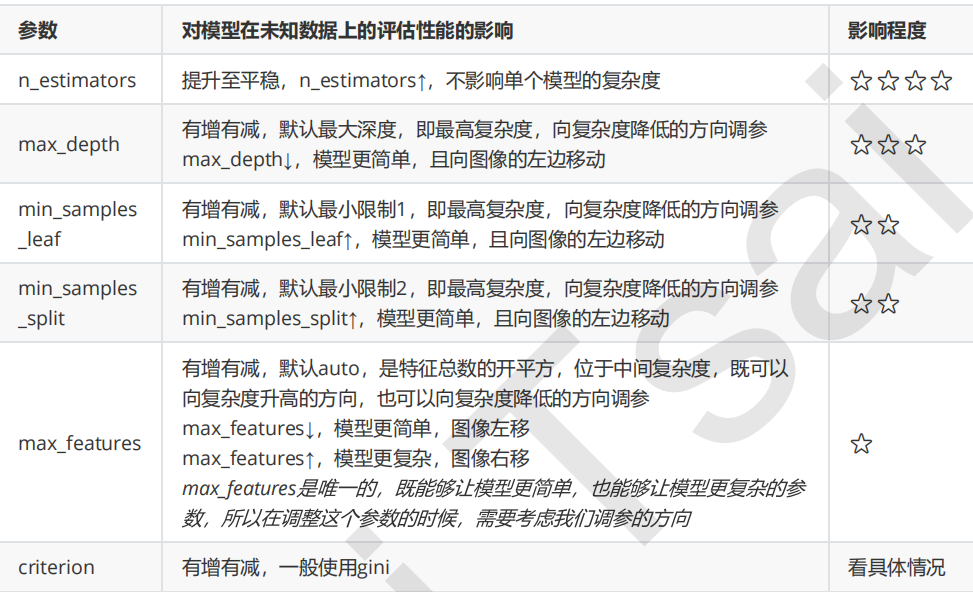#十一、乳腺癌数据上的调参

rf = RandomForestClassifier(random_state=10)
score = cross_val_score(rf,cancer.data,cancer.target,cv=10).mean()
score


#n_estimators的学习曲线  确定大致范围  间隔是10
scores = []
for i in range(0,200,10):
rf = RandomForestClassifier(n_estimators=i+1,random_state=10)
score = cross_val_score(rf,cancer.data,cancer.target,cv=10).mean()
scores.append(score)
print(max(scores))
plt.plot(range(1,201,10),scores)
plt.show()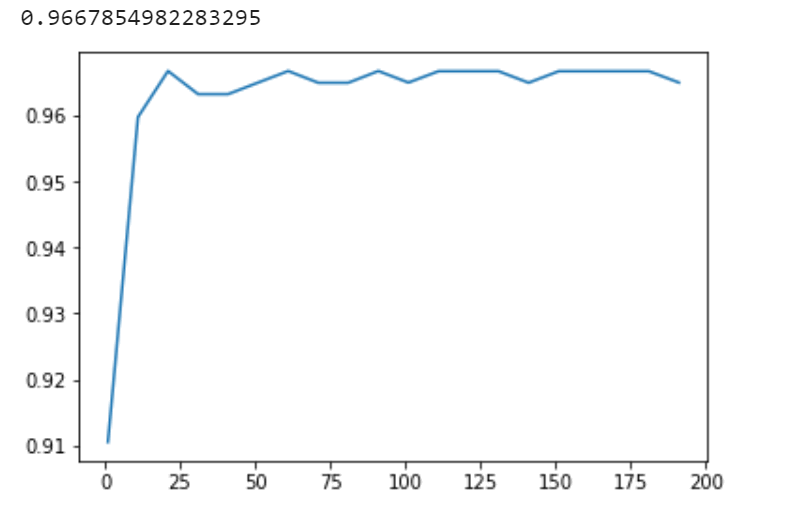#n_estimators的学习曲线  最终确定
scores_2 = []
for i in range(10,25):
rf = RandomForestClassifier(n_estimators=i+1,random_state=10)
score = cross_val_score(rf,cancer.data,cancer.target,cv=10).mean()
scores_2.append(score)
print(max(scores_2),scores_2.index(max(scores_2))+10+1)
plt.plot(range(11,26),scores_2)
plt.show()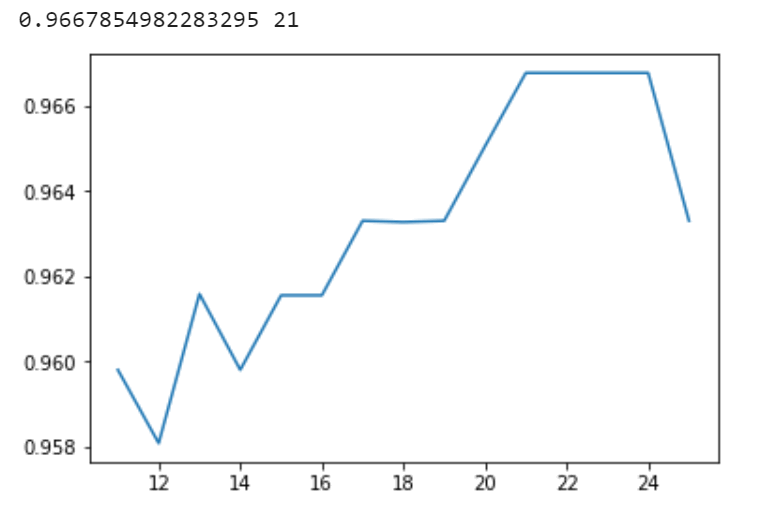#耍一下网格搜索，其实一般是不大好进行预测的时候用
from sklearn.model_selection import GridSearchCV
grid_params = {"max_depth":np.arange(1,11,1)}
rf = RandomForestClassifier(n_estimators=21,random_state=20)
grid = GridSearchCV(rf,grid_params,cv = 10)
grid.fit(cancer.data,cancer.target)
print(grid.best_params_,grid.best_score_)


05-16948108-233825
02-02378
11-23202
10-189232
05-145197
04-224808
07-24570
09-09189
01-15
05-2518万+
01-272045
02-08226
11-201169
03-081721
09-14857
04-156万+
03-141万+
09-202512点击重新获取扫码支付余额充值